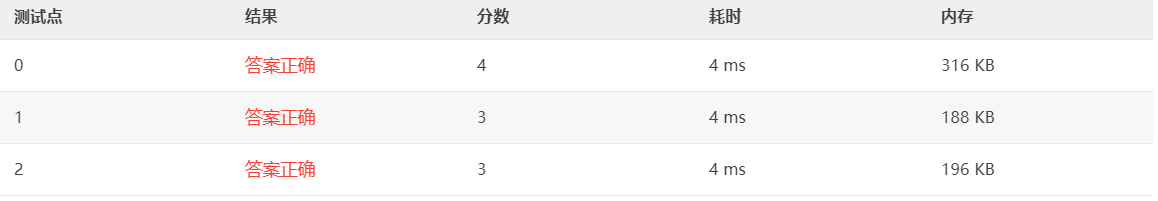signed

QiShunwang

“诚信为本、客户至上”

# 7-7 通讯录排序 (10 分)

2021/4/26 23:11:28   来源：

### 输入样例:

``````3
zhang 19850403 13912345678
wang 19821020 +86-0571-88018448
qian 19840619 13609876543``````

### 输出样例:

``````wang 19821020 +86-0571-88018448
qian 19840619 13609876543
zhang 19850403 13912345678``````
`````` #include<stdio.h>
using namespace std;
struct friends{
char name;
int birthday;
char number;
}a;
int main(){
int n;scanf("%d",&n);

for(int i=0;i<n;i++ )
{
scanf("%s",&a[i].name);
scanf("%d",&a[i].birthday);
scanf("%s",&a[i].number);
}
for(int j=0;j<n;j++){
for(int i=0;i<n-j-1;i++){
if(a[i].birthday>a[i+1].birthday){
struct friends b=a[i];
a[i]=a[i+1];
a[i+1]=b;
}

}
}
for(int i=0;i<n;i++){
printf("%s %d %s",a[i].name,a[i].birthday,a[i].number);
printf("\n");
}

return 0;
}
``````冒泡排序法老是出错....还是得加深印象。